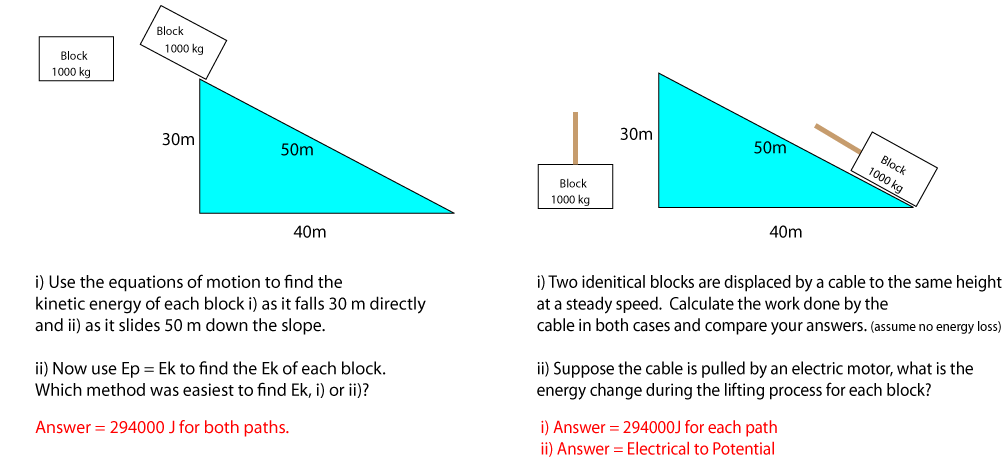## Learning Outcomes

Aids to Understanding

International System of Units

The second, kilogram and meter are called SI units (International System or Systčme International d'unités) There are seven units in total. The units are often prefixed by a term that denotes multiples of thousandth or thousands. For example a millisecond is one thousandth or a second and a microsecond is one thousand thousandth (millionth) of a second These terms are precisely measured. For example the actual mass of a kilogram is almost exactly equal to the mass of 1 litre of water and its prototype lies in a vault in International Bureau of Weights and Measures in France. The metre is defined as the distance light travels through a vacuum 1/299,792,458 of a second. (We clearly know the speed of light very acurately). The second is defined to be the duration of 9,192,631,770 periods of the radiation corresponding to the radiation produced during an electronic transition between the two hyperfine levels of the ground state of the caesium 133 atom. We will appreciate this meaning when we have studied Unit 3.

Note: A point about Labelling Force Vectors

If we have a a cart being pulled by a horse, then the the force vector is labelled FCH, however in the free body diagram of the cart it is acceptable to write FH; the body diagram indicates the body experiencing the force. Example: Draw a free body diagram for ..
i) a log floating on water
ii) a helicopter beginning to rise
iii) a ball freely falling
iv) a ball freely rising
(Note: iii) and iv) have identical body diagrams)

Analysing the Lift

The motion of a lift is controlled by the cable attached to it. This cable can exert a force on the lift (FLC)that is ..

1. equal to to weight of the lift
2. greater than the weight of the lift
3. less than he weight of the lift

However the lift can have the following motions

1. the lift can be at rest
2. the lift can pick up speed going up
3. the lift can travel upwards at a uniform (steady) speed
4. the lift can slow down going up
5. the lift can pick up speed going down
6. the lift can travel downwards at a uniform speed
7. the lift can slow down going down

So we have three different cable tensions enabling seven different stages of a lift's motion.

Problem:

i) Draw a free body diagram for a lift of mass 3000 kg for each of the seven stages. Assume the (FLC) (aka Tension) can be no more than 35000 N or no less than 25 000 N and use these accordingly.
ii) Find the accelerations of the lift and find the reading on Newton scales if a woman of 50 kg is standing on them at each of the seven stages.

Forces Down Slopes

A block on a slope will accelerate down the slope but clearly the acceleration is less than -9.8 ms-2. To find the force on the block down the slope due to the Earth, we draw a block of mass m on a slope of angle q. We draw in the Force on the block due to the Earth, resolve this force into two rectangular components, one of which is parallel to the slope (blue). Put these vectors head to toe, use simple trigonometry and you will find the force on a block down a slope = mgSinq.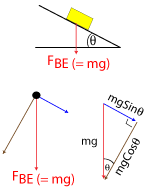## 1) The unit of Force: The Newton.

The fundamental (simplest) units in the physics of motion are kilograms (kg), metres (m) and seconds (s). This means that these quantities can be defined using one measurement only. For example the second is an agreed period of time, the kilogram is an agreed mass and the metre is an agreed length. The units of speed (ms-1) and acceleration (ms-2)on the other hand are made up using units of length (m) and time (s). The unit of force is not fundamental, is called the Newton and it is made up of units of length, time and mass. If a unbalanced force is acting on a body of 1 mass kg and its acceleration is 1 ms-2, then the unbalanced force is equal to 1 Newton 1 N. This is the definition of 1 Newton.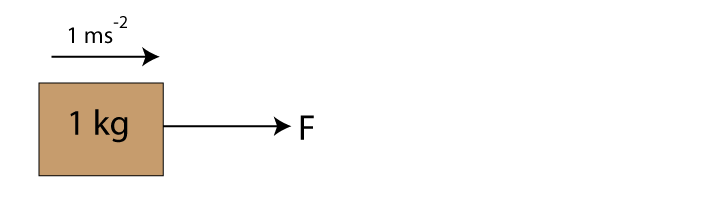Given a 1N unbalanced force acting on a 1 kg mass will cause the mass to accelerate by 1 ms-2, fill in the blanks below and find an expression for F, m and a,
Unbalanced Force (N) Mass (kg) Acceleration (ms-2)
2 1 ?
4 ? 4
? 4 2
The answers are 2ms-2, 1 kg, and 8 N, respectively. You should see from this that the definition of Newton, leads to F = ma. This is called Newton's second law and we discovered it empirically (by experiment) is S4.

## 2) Free body Diagrams

Free body diagrams simplify force problems and are powerful analalytical tools. Free body diagrams do not indicate infromation about speed, they do, however yeild information on acceleration. The context alone informs us of the direction of the velocity and whether or not it is increasing or decreasing. The table illustrates free body diagrams for some situations you may come across in higher physics. Note: Free body diagrams are so called because when analysing the forces on a body we completely isolate (or free) the body from its surroundings and focus on the forces acting on the body, alone. Only these forces appear on our free body diagrams.
Look at starred * rows for the car and ** rows for the parachutist, notice we have identical free body diagrams but the motion is different in each case.
Direction of velocity Nature of motion Free body diagram free body diagram showing Resultant Force only(N) Accel = Fr/m (ms-2)
Velocity to the Right A car is travelling at a steady speed of 3ms-1.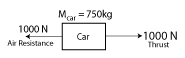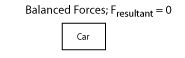0
Velocity to the Right A car is getting faster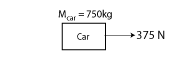0.5
*Velocity to the right A car is slowing down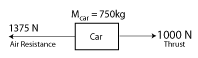-0.5
*Velocity to the left The car is speeding up-0.5
Velocity of skydiver is always downwards (negative) A skydiver is travelling at -20ms-1 and getting faster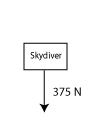5
**Velocity of skydiver is always downwards (negative) The skydiver is falling at a steady speed of 60ms-1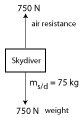0
Velocity of skydiver is always downwards (negative) The skydiver has pulled the ripcord40
**Velocity of skydiver is always downwards (negative) The skydiver is falling with a gentle constant speed of 5 ms-1>0

#### Labelling Force Vectors

When we are drawing force vectors we must be aware of what is causing the force, what the force is acting on, whether the force is a reaction or reaction force, whether the force is balanced or unbalanced and the direction of the force. Free body diagrams and effective labelling of forces vectors aid clear thinking about the nature of force. Suppose A is pulling on B with a Force F. How do we comprehensively but precisely express this statement? Simple.just write FAB = Force on A due to B. This is the convention we use when labelling force vectors. Notice the body the force acts on (A) is listed first.
Situation Force Notation How it is read Action or Reaction?
Lift pulled vertically by cable FLC Force on Lift due to Cable Action
Man pulled by Earth FME Force on Man due to Earth Action
Man pushed up by scales FMS Force on Man due to Scales Reaction
Apple supported by balance FAB Force on Apple due to Balance Reaction
Tug boat pulling an oil Rig FRT Force on Rig due to Tugs Action
Sprinter pushed by ground FSG Force on Sprinter due to Ground Reaction

## 3) Unbalanced Force = Mass x Acceleration - Newton's Second Law in Context

A common problem in higher physics involves motion in a lift. Very often the problem involves a person standing on scales, or a box hanging from a newton balance. The question usually asks what the reading on the scales will be.
Example: A lift and contents has a mass of 2000 kg. If the lift experiences an upwards force (Tension) of 22000 N from a cable, calculate
1. The acceleration of the lift.
2. The reading on the Newton balance when a 160 g apple is hanging from it.
3. The reading on the scales when an 80 kg man is standing on them.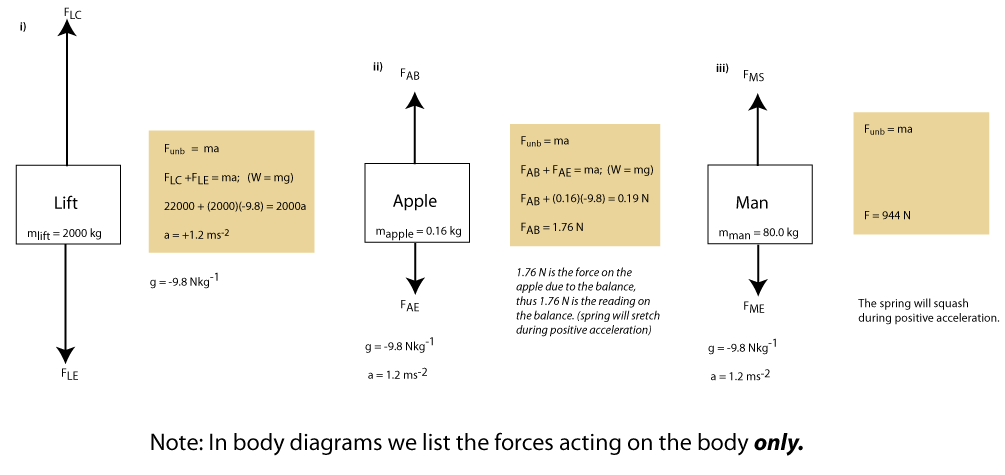## 4) Energy calculations in Mechanics

Whenever a force is acting on a body and causes a displacement of that body, then the force is transferring energy to the body (or its surroundings) and we say the force is doing work. Work Done (by the force) is therefore an energy measured in Joules (J) and it is defined as Force (N) x Displacement (m). Ew = F.s (J). 1 J = 1 Nm.#### Work Done = kinetic Energy and Potential Energy

The three expressions for energy and one for power are shown below. You should be familiar with them from S4.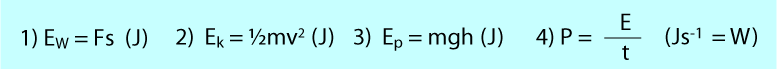The Work Done by a force on a body is the energy transferred to the body. In mechanics the energy the body acquires can manifest itself as kinetic energy, potential energy or heat energy. The following argument shows that Work Done on a body equals its change in Kinetic Energy. (provided there are no other forces present).
As you read this argument draw a diagram to support it. Suppose a block is at rest and u = 0. Now suppose a uniform force pulls (Works) the block until its velocity is v. If this change in velocity occurred in a time t, then the average velocity of the block is v/2 and its acceleration is v/t. If the block was displaced s metres during this time then the average velocity of the block is s/t. Thus s/t = v/2 or s = vt/2. Now Ew = Fs = mas = m(v/t)(vt/2) = 1/2mv2. This Ew = Ek. Suppose the block was not initially at rest, show that Ew = DEk.
Exercise: Draw a diagram to support this argument and using sensible values verify that Ew = DEk.
A similar argument can be used to show Ew = Ep. Suppose a Force F, slowly (therefore we can ignore Ek lift a block m a height h. Then we can say Ew = Fs = (mg)h = mgh.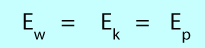## Cumulative problem

These questions will help you appreciate the energy expressions and how they can be used to simply problems. Note in these questions we ignore friction.